# Trigonometry Without Pain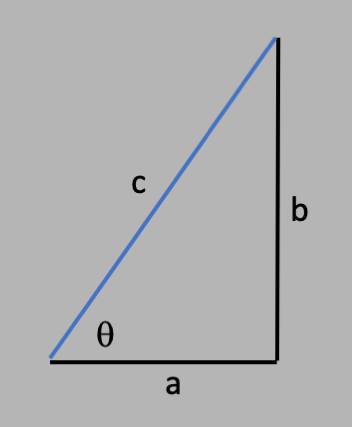## Introduction to Trigonometry

Back to top

Trigonometry is one of those subjects that will either bore you to tears, drive you crazy, or fascinate you. Either way, the bottom line is that trigonometry was discovered because it's useful, and has a purpose. And that means everyone can understand it if they start at the beginning and work their way through it.

So, the beginning of trigonometry starts with the intersection of 2 lines, and in particular, when those 2 lines cross at right angles:The right angle is very special, because when lines cross at right angles, the lines are completely independent. That is, you can walk along either line and get nowhere along the other line. Try crossing 2 lines at an angle other than a right angle, and if you walk along one of the lines, you will slowly walk along the other. That's what it means to be independent.

Now, if you connect the two open ends of the lines, that connection is called the "hypotenuse", which comes from the Greek word "stretch":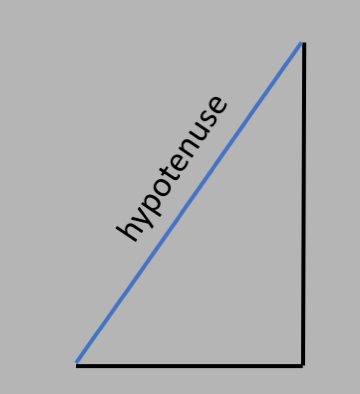Using more Greek letters, we define the angle $\theta$ like so: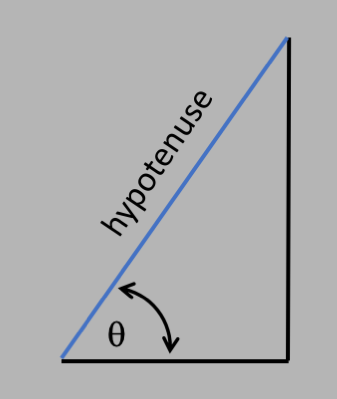We could have defined $\theta$ using the angle between the hypotenuse and the vertical line, but it doesn't matter, as we will see later.

Trigonometry, and all of the formulae that drive you crazy (or inspire you) involve understanding the relationship between all of the lengths, and the angle $\theta$:Figure 1. Right triangle.

So we have 3 lengths, and 1 angle, so why don't we care about the other 2 angles inside the triangle? To understand this, first let's discuss how we measure angles, and to do that we first have to consider the circle. In the picture below, we have 2 lines that intersect at right angles (black), and a ray that points from the center outward (blue). The ray makes an angle $\theta$ with the horizontal, and when you go around one full turn, you get back to the same angle that you started with, as in the figure below: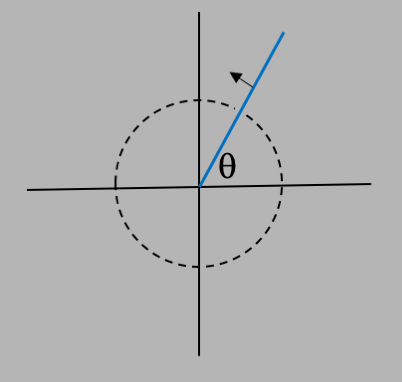So we could define the angle $\theta$ as being between 2 limits, e.g. $0$ and $1$, or we can use some other measure. Thanks to the early Mesopotamian mathematicians, who used a number system in base 60, instead of $0\le\theta\le 1$ we say that $0\le\theta\le 360$ degrees, where $\theta=0$ is the same angle as $\theta=360\deg$, where the symbol $\deg$ means "degrees". Given this measure, we now can see that a right angle is $360/4=90\deg$, and halfway around is $180\deg$.

Now back to the right triangle, and the question about why we are only considering one of the 3 internal angles (and the 3 sides): the reason is that we already know the right angle, which is $90\deg$, and in fact the sum of all the angles is equal to $180\deg$. For the proof, let's label the other angle between the hypotenuse and the vertical line as $\phi$, and then make a copy of the triangle but flip it over so that the vertical line is to the left, and the horizontal line is on the top. And then attach the 2 triangles at the hypotenuse, as in the following figure: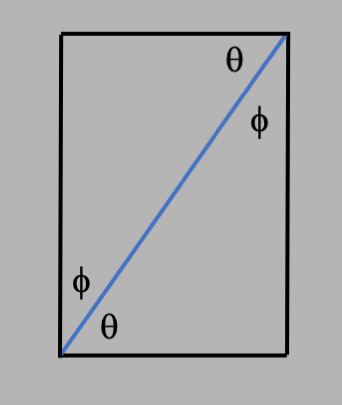Since we've flipped the triangle over, the 2 angles $\theta$ and $\phi$ are the same on both sides. Now, since the figure becomes a rectangle, then all of the interior angles of a rectangle are right angles ($90\deg$), and since there are 4 of them, they sum to $360\deg$. But since the rectangle is made of 2 identical triangles, then the sum of the interior angles of the triangle is $180\deg$, which means $\theta + \phi + 90\deg = 180\deg$ or $\theta + \phi = 90\deg$. So if we know $\theta$, we know $\phi$, they are not independent, and that's why we are only worrying about $\theta$ here. (Or as they say in geometry: QED).

Now to make things even more complicated, let's consider any angle $\theta$ that defines an arc of a circle as in the figure below, where $r$ is the radius of the circle and $s$ is the length of the arc: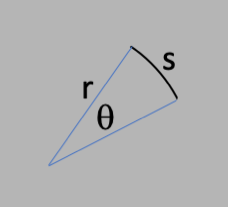What is the ratio of $r/s$? Well, one thing we know from the ancients is that if $\theta = 360\deg$, then the ratio of the circumference $s$ (the arc length of the complete circle) to the diameter $d=2r$ is $s/2r=\pi$, or $s\r=2\pi$ where $\pi=3.14159...$. This implies that we can measure angles in units (called "radians") such that $360\deg = 2\pi$ radians or $180\deg = \pi$, which is how we convert from degrees to radians. And this also says that the ratio of the arc length to the radius tells you the value of the angle, but only if you measure it in radians!

So now we know how to measure angles, but we want to go back to trigonometry, which starts with right triangles. For our triangle in Figure 1, we want to investigate the relationship between the 3 sides and the interior angle $\theta$. From Pythagoras, we have the Pythagorean theorem, which states that $$c^2 = a^2 + b^2\nonumber$$ which means that in fact we only have 2 independent lengths.

So now we are ready to learn about trigonometry and the trig functions. Since only 2 of the 3 lengths are independent, we can ask about the relationship between any two lengths. So let's keep $a$ constant, let $b$ vary, and require that the triangle stay a right triangle, which forces $a^2 + b^2 = c^2$ by the Pythagorean theorem. In the figure below you can see two examples. Notice that as $b$ gets larger, so does $\theta$. The question is: how does $\theta$ change when you change $b$?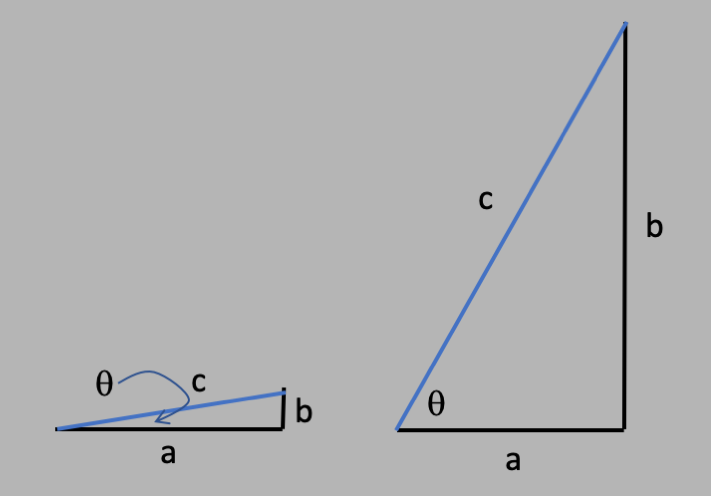Figure 1.

To understand this, let's take the ratios $a/c$ and $b/c$. If we use the Pythagorean theorem, we can easily show that $$\Big(\frac{a}{c}\Big)^2 + \Big(\frac{b}{c}\Big)^2 = 1\nonumber$$ And clearly, as the ratios change, so will the angle $\theta$ change. So we can define these ratios in the following way. (Note that the word "sine" is named after the Greek word "sine" which means "curve".)

• "Sine": $\sin\theta =$ opposite/hypotenuse
• "Cosine": $\cos\theta =$ adjacent/hypotenuse

In the figure below, we plot the ratios (sine and cosine) as a function of angle, with sine in red and cosine in blue.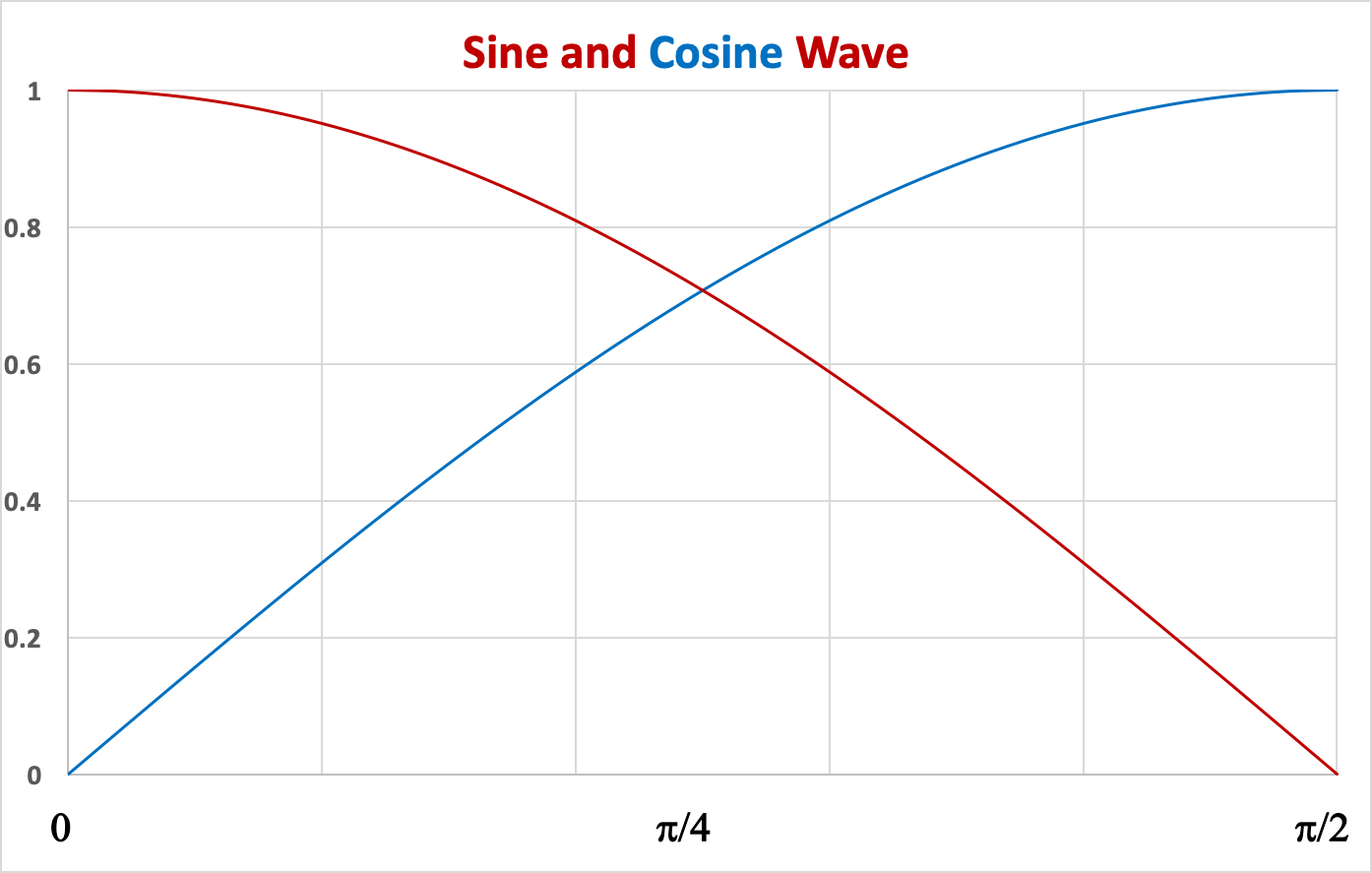Figure 2.

The plot shows you $\sin(\theta)$ on the vertical axis in blue, and $\cos(\theta)$ on the vertical axis in red, vs $\theta$ on the horizontal, where $\theta$ is measured in radians. When $\theta=0$, that corresponds to a very small ratio of $b/c$, like the left triangle in Figure 1 above. In that case, when the opposite/hypotenuse is small, the adjacent/hypotenuse is large, as in the the right triangle in Figure 1. Since the adjacent/hypotenuse is the cosine, then for $\theta=0$ we expect the cosine go be at 1, as it is in the red curve above. You can make similar arguments for the case of $\theta=90\deg=\pi/2$, which has $b/c$ close to $1$, so opposite/hypotenuse (sine) goes to $1$ and adjacent/hypotenuse (cosing) goes to $0$.

What you can also see from this diagram is that $\cos(\pi/2-\theta)=\sin(\theta)$ (and by the same argument, $\sin(\pi/2-\theta)=\cos(\theta)$). More on that below.

So now we know how the sine and cosine are defined, and how they relate to the lengths of the adjacent and opposite sides of a triangle (adjacent and opposite to the angle $\theta$). But we've only defined these 2 functions for the situation where $\theta$ is between $0$ and $\pi/2$. What about the other 3/4 of a circle: $\pi/2\le\theta\le 2\pi$? To extend the definitions over the entire range of $\theta$, we introduce the concept of "the unit circle", as in the figure below: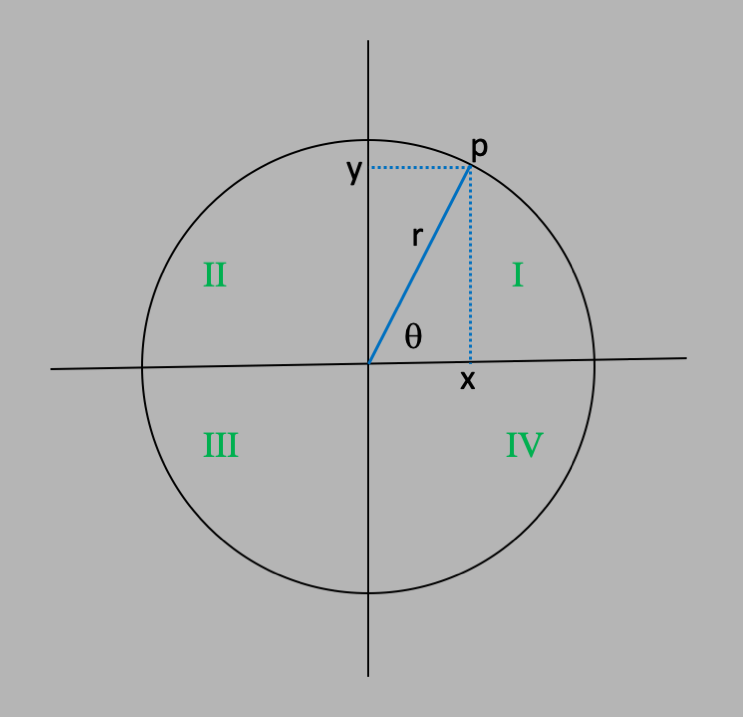We define the angle $\theta$ in the same way as above: the angle that a ray with one end at the origin makes with the horizontal. In this figure, the circle has a radius $r$ and is centered at the origin, so that no matter what $\theta$ is, the ray touches the circle (circles are defined as the locus of all points that have a constant distance from some fixed origin). In this circle, we can choose the length $r$ so we choose $r=1$ to make things easier below. The point $p$ is the intersection of the ray with the circle. Notice that the ray forms a right triangle with adjacent side $x$ (instead of $a$) and opposite side $y$ (instead of $b$). The definition of the sine and cosine still hold here, however instead of saying that the sine is the ratio of opposite/hypotenuse, we say that the sine tells you the value of the position along the horizontal when you draw a perpendicular line connecting point $p$ to the horizontal line. So in this figure, $x$ is a coordinate along the horizontal axis, and can be positive ($x$ to the right of the vertical line) or negative ($x$ to the left). And similarly for y, which is the coordinate along the vertical of the perpendicular line from $p$ to the vertical axis. Note that $\sin\theta=y/r$ (opposite/hypotenuse) and $\cos\theta=x/r$ (adjacent/hypotenuse), but since $r=1$, we can write $\sin\theta=y$ and $\cos\theta=x$. Also, in green we have labeled the 4 quadrants of the circle, starting in the upper right quadrant and increasing as we go around counterclockwise. This will be used below.

Now we can define sine and cosine by saying that no matter where $\theta$ is on the circle, if we drop perpendicular lines from the point $p$ to the vertical and horizontal axes, we will have the value for the $\sin\theta$ and $\cos\theta$, anywhere on the circle. Cute eh? In the figure below, you can click on the solid red circle and drag it around the blue (unit) circle and see how $\theta$ changes, and the projected $x$ and $y$ coordinates (given by $\sin\theta$ and $\cos\theta$) move.

 $\theta$ $=$ 45$\deg$ $\sin\theta$ $=$ 0.707 $\cos\theta$ $=$ 0.707

We now have a way of defining the sine and cosine functions over the full cycle $0$ to $2\pi$ ($0$ to $360\deg$): they represent the coordinates of points on the unit circle.

Notice that all 4 quadrants of the unit circle are pretty much equivalent, with the only difference being that some are reflections of others. For instance, quadrant I (where $x$ and $y$ are both positive) is similar to quadrant II (where $x$ is negative but $y$ is still positive), but flipped over the vertical axis. So we would expect that the graph of $\sin\theta$ between $90\deg$ and $180\deg$ is similar to the graph of $\sin\theta$ between $0\deg$ and $90\deg$, except that in the latter as $\theta$ increases, $\sin\theta$ increases over the interval and in the former, it decreases. So it should be relatively easy to construct the curve of $\sin\theta$ vs $\theta$ over the entire interval using the curve in quadrant I as in Figure 2. above. In the figure below, we see the sine curve over the entire range of $\theta$ between $0$ and $2\pi$ radians. Notice the symmetry in the 4 quadrants: in quadrant I, $\sin\theta$, the $y$ coordinate, increases from 0 to 1 whereas in quadrant II, the $y$ coordinate decreases from 1 to 0. In quadrant III, the $y$ coordinate decreases from 0 to -1 and in quadrant IV, it increases from -1 to 0. So we could use the curve in Figure 2 and draw the entire sine curve over the full interval $0\le\theta\le 2\pi$, as in the figure below: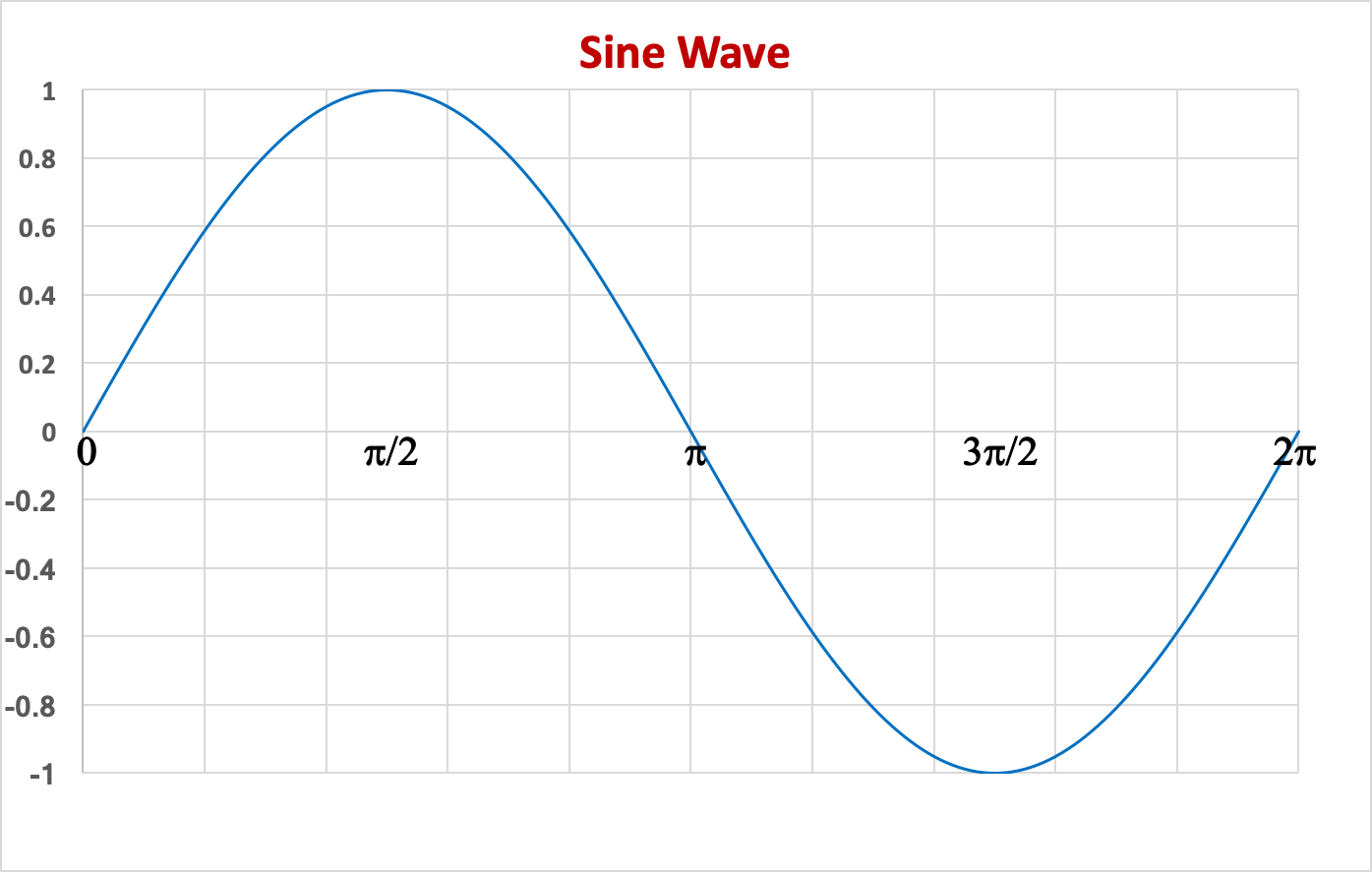Similarly, we can draw the full cosine curve in the same interval: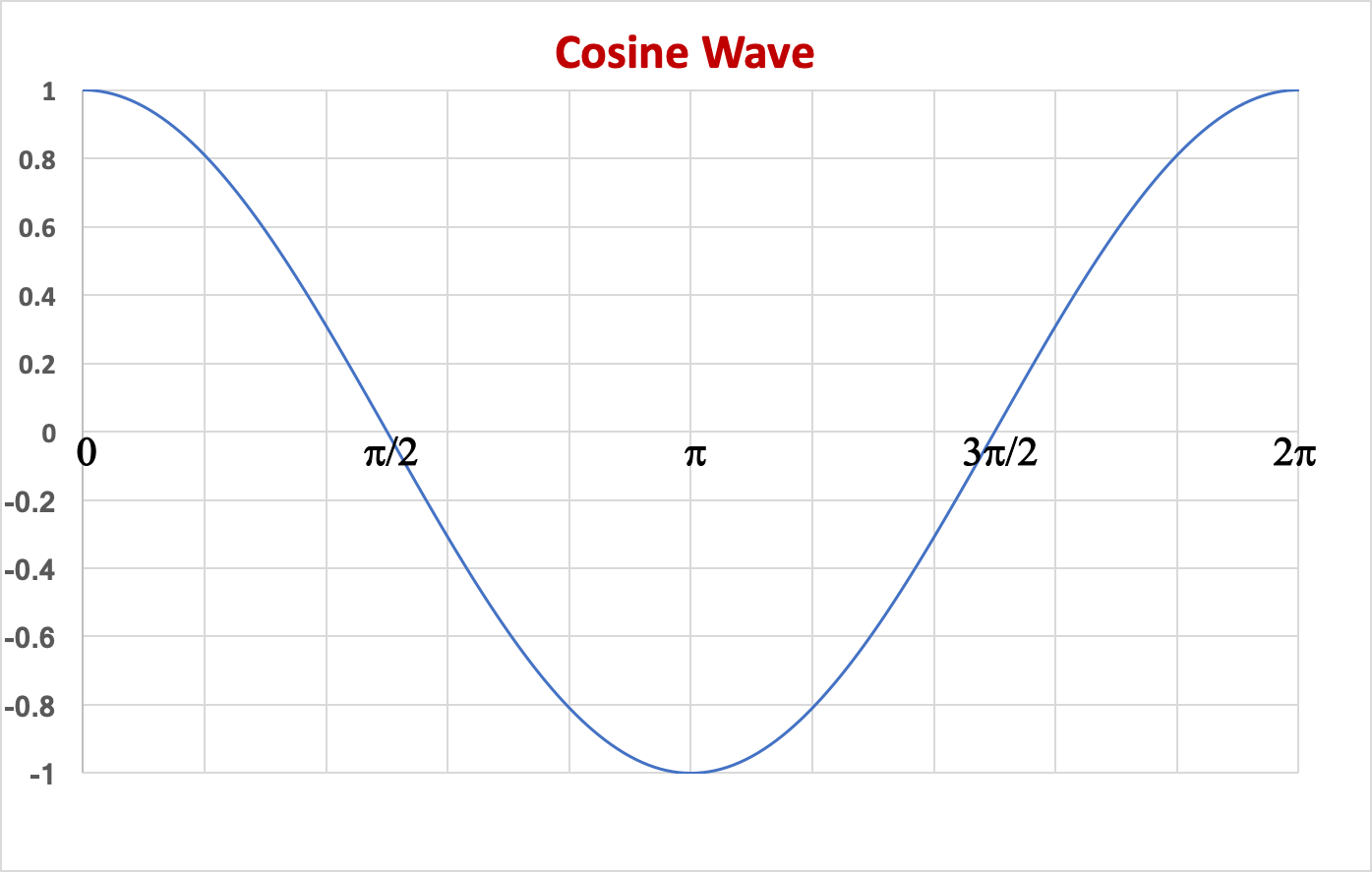One last note: above, we showed that since the interior angles of a right triangle add up to $180\deg$, that means $\theta +\phi=90\deg$, and that means that $\sin(\pi/2-\theta)=\cos(\theta)$. Graphically, this means that if you take the sine curve and move it to the left by $\pi/2$, you will get the cosine curve. And voila, that's what the 2 curves above show!

## More Trig Functions

Back to top

Now that we have the sine and cosine functions as the ratios of the opposite and adjacent lengths to the hypotenuse, we can form the remaining trig functions. The table below has all 6 trig functions:

 Sine $\sin\theta$ opposite/hypotenuse Cosine $\cos\theta$ adjacent/hypotenuse Tangent $\tan\theta$ $\sin\theta/\cos\theta$ opposite/adjacent Cotangent $\cot\theta$ $1/\tan\theta$ adjacent/opposite Secant $\sec\theta$ $1/\cos\theta$ hypotenuse/adjacent Cosecant $\csc\theta$ $1/\sin\theta$ hypotenuse/opposite

## Properties of Trig Functions

Back to top

Now that we have all of the 6 trig functions, we can explore properties. For instance, the Pythagorean theorem relates the 3 sides of the triangle $a$, $b$, and $c$ (where $c$ is the hypotenuse): $$a^2 + b^2 = c^2\nonumber$$ This also tells us that $$\sin^2\theta + \cos^2\theta = 1\label{fm}$$ and that this relationship is true no matter what value $\theta$ takes on. Note that $\sin^2\theta$ means the same as $(\sin\theta)^2$, and not $\sin\theta^2$.

If we take equation $\ref{fm}$ and divide each side by $\cos^2\theta$, we get: $$\tan^2\theta + 1 = \sec^2\theta\nonumber$$ If we take equation $\ref{fm}$ and divide by $\sin^2\theta$, we get: $$1 + \cot^2\theta= \csc^2\theta\nonumber$$ Another useful property of the sine and cosine has to do with their "symmetry". That is, what is $\sin(-\theta)$ and $\cos(-\theta)$. There are many ways to think of how to do this, but one useful way to think of this is to realize that a negative angle is the same as $360\deg$ minus that angle (e.g. $-90\deg$ is the same as $270\deg$). And since we now know that the $\sin$ and $\cos$ are the same things as the $y$ and $x$ coordinates of the point on the unit circle respectively, if you go to negative angle then the $y$ coordinate changes sign and the $x$ coordinate stays the same, as in the figure below: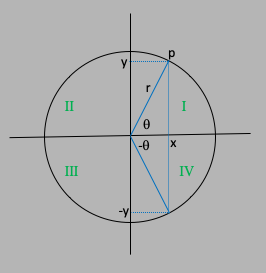So this says that we have the relations: $$\sin(-\theta) = -\sin(\theta)\label{esn}$$ $$\cos(-\theta) = +\cos(\theta)\label{ecn}$$

## Sine and Cosine of Sums of Angles

Back to top

We often want to take the sine or cosine of a sum of angles, e.g. $\sin(\theta + \phi)$ and $\cos(\theta + \phi)$. Below is a nice way to derive formulae on how to do calculate the sine and cosine of sums using the sine and cosines of the individual angles.

We start with the figure below, which sets up the proof. We want to calculate $\sin(\theta + \phi)$, so we draw a rectangle (with 4 right angles), and inscribe a right triangle inside the square in blue. The hypotenuse of that triangle is set to 1 by fiat: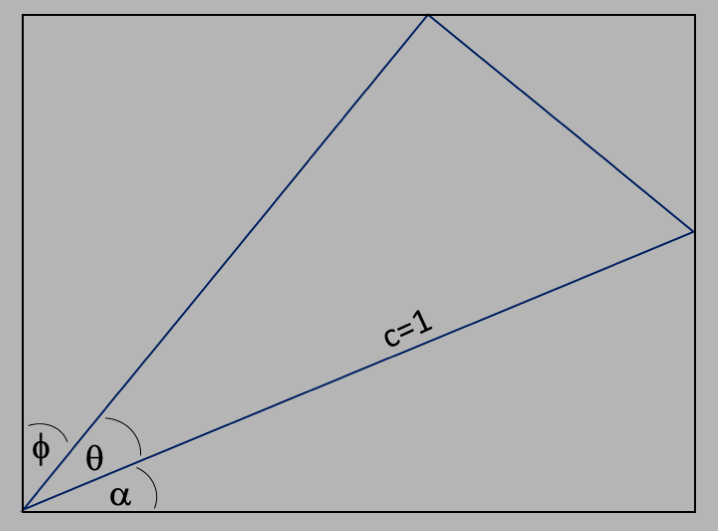The angle $\alpha$ is also shown, but since $\alpha$, $\theta$, and $\phi$ form a right triangle, we know that $\alpha = 90\deg - \theta - \phi$. And since $\alpha$ forms one of the angles of the right triangle that has the blue hypotenuse and the horizontal bottom of the rectangle as a base, we also know that the other angle of that triangle is $\theta + \phi$, as in the figure below:Using the fact that the hypotenuse is set to 1, and the definitions of the sine (opposite/hypotenuse) and cosine (adjacent/hypotenuse), we can then label the lengths of the 2 sides of the inscribed triangle as in the next figure: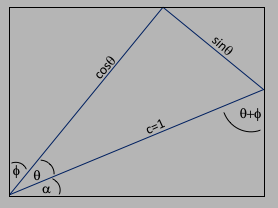Now let's calculate $\sin(\theta+\phi)$, which is the length of the bottom horizontal leg of the rectangle, as labeled in the figure below. Also, we've labeled the interior angle $\beta$, which has a value $\beta = 90\deg - \phi$ and since $\beta$ and the right angle of the inscribed triangle and the interior angle (marked $\phi$ at the top) add up to $180\deg$, that means that the angle $\phi$ at the top is the same angle $\phi$ in the bottom left corner. And we've labeled the two legs of the upper side of the rectangle as $a$ and $b$.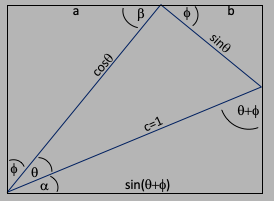Now we are ready to calculate the two legs of the upper side of the rectangle. The length $a$ is related to the angle $\phi$ by the ratio of opposite to hypotenuse, so $a=\sin\phi \cdot \cos\theta$, and the length $b$ is related to the angle $\phi$ by the ratio of adjacent to hypotenuse, so $b=\cos\phi\cdot\sin\theta$. From the equivalence of the upper to the lower rectangle side, we have $$\sin(\theta+\phi) = a + b\nonumber$$ or $$\sin(\theta+\phi) = \sin\phi\cos\theta + \sin\phi\cos\theta\nonumber$$ Using similar logic on the two vertical sides of the triangle, we can equate the left side - $\cos\phi\cos\theta$ - to the sum of the two legs on the right side - $\sin\phi\sin\theta + \cos(\theta+\phi)$ - to get the relation:\ $$\cos(\theta+\phi) = \cos\phi\cos\theta - \sin\phi\sin\theta\nonumber$$ To calculate $\sin(\theta-\phi)$ and $\cos(\theta-\phi)$ we can use equations $\ref{esn}$ and $\ref{ecn}$ and subsitute $\phi\to\-\phi$ to get: $$\sin(\theta-\phi) = \sin\phi\cos\theta - \sin\phi\cos\theta\nonumber$$ and $$\cos(\theta-\phi) = \cos\phi\cos\theta + \sin\phi\sin\theta\nonumber$$ So the final equations are: $$\sin(\theta\pm\phi) = \sin\phi\cos\theta \pm \sin\phi\cos\theta\label{es2}$$ and $$\cos(\theta\pm\phi) = \cos\phi\cos\theta \mp \sin\phi\sin\theta\label{ec2}$$ Equations $\ref{es2}$ and $\ref{ec2}$ are used quite a lot in trigonometry! For instance, we can set $\phi=\theta$ and use eqation $\ref{es2}$ (the positive one) to calculate: $$\sin 2\theta = 2\sin\theta\cos\theta\label{es2t}$$ and $$\cos 2\theta = \cos^2\theta - \sin^2\theta\label{ec2t}$$ If we combine equations $\ref{ec2t}$ and $\ref{fm}$, we get $$\cos^2\theta = \frac{1+\cos 2\theta}{2}\label{ec22t}$$ And since $\cos^2\theta+\sin^2\theta = 1$, we have $$\sin^2\theta = \frac{1-\cos 2\theta}{2}\label{es22t}$$